# Excel INDEX MATCH MATCH and other formulas for two-way lookup

The tutorial showcases a few different formulas to perform two dimensional lookup in Excel. Just look through the alternatives and choose your favorite :)

When searching for something in your Excel spreadsheets, most of the time you'd look up vertically in columns or horizontally in rows. But sometimes you need to look across both rows and columns. In other words, you aim to find a value at the intersection of a certain row and column. This is called matrix lookup (aka 2-dimensional or 2-way lookup), and this tutorial shows how to do it in 4 different ways.

## Excel INDEX MATCH MATCH formula

The most popular way to do a two-way lookup in Excel is by using INDEX MATCH MATCH. This is a variation of the classic INDEX MATCH formula to which you add one more MATCH function in order to get both the row and column numbers:

INDEX (data_array, MATCH (vlookup_value, lookup_column_range, 0), MATCH (hlookup value, lookup_row_range, 0))

As an example, let's make a formula to pull a population of a certain animal in a given year from the table below. For starters, we define all the arguments:

• Data_array - B2:E4 (data cells, not including row and column headers)
• Vlookup_value - H1 (target animal)
• Lookup_column_range - A2:A4 (row headers: animal names) - A3:A4
• Hlookup_value - H2 (target year)
• Lookup_row_range - B1:E1 (column headers: years)

Put all the arguments together and you will get this formula for two-way lookup:

`=INDEX(B2:E4, MATCH(H1, A2:A4, 0), MATCH(H2, B1:E1, 0))`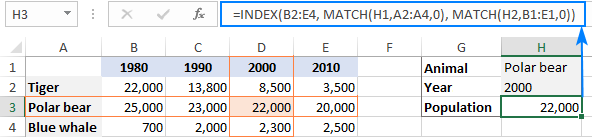If you need to do a two-way lookup with more than two criteria, take a look at this article: INDEX MATCH with multiple criteria in rows and columns.

### How this formula works

While it may look a bit complex at first glance, the formula's logic is really straightforward and easy to understand. The INDEX function retrieves a value from the data array based on the row and column numbers, and two MATCH functions supply those numbers:

`INDEX(B2:E4, row_num, column_num)`

Here, we leverage the ability of MATCH(lookup_value, lookup_array, [match_type]) to return a relative position of lookup_value in lookup_array.

So, to get the row number, we search for the animal of interest (H1) across the row headers (A2:A4):

`MATCH(H1, A2:A4, 0)`

To get the column number, we search for the target year (H2) across the column headers (B1:E1):

`MATCH(H2, B1:E1, 0)`

In both cases, we look for exact match by setting the 3rd argument to 0.

In this example, the first MATCH returns 2 because our vlookup value (Polar bear) is found in A3, which is the 2nd cell in A2:A4. The second MATCH returns 3 because the hlookup value (2000) is found in D1, which is the 3rd cell in B1:E1.

Given the above, the formula reduces to:

`INDEX(B2:E4, 2, 3)`

And return a value at the intersection of the 2nd row and 3rd column in the data array B2:E4, which is a value in the cell D3.

## VLOOKUP and MATCH formula for 2-way lookup

Another way to do a two-dimensional lookup in Excel is by using a combination of VLOOKUP and MATCH functions:

VLOOKUP(vlookup_value, table_array, MATCH(hlookup_value, lookup_row_range, 0), FALSE)

For our sample table, the formula takes the following shape:

`=VLOOKUP(H1, A2:E4, MATCH(H2, A1:E1, 0), FALSE)`

Where:

• Table_array - A2:E4 (data cells including row headers)
• Vlookup_value - H1 (target animal)
• Hlookup_value - H2 (target year)
• Lookup_row_range - A1:E1 (column headers: years)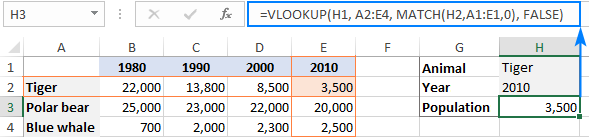### How this formula works

The core of the formula is the VLOOKUP function configured for exact match (the last argument set to FALSE), which searches for the lookup value (H1) in the first column of the table array (A2:E4) and returns a value from another column in the same row. To determine which column to return a value from, you use the MATCH function that is also configured for exact match (the last argument set to 0):

`MATCH(H2, A1:E1, 0)`

MATCH searches for the value in H2 across the column headers (A1:E1) and returns the relative position of the found cell. In our case, the target year (2010) is found in E1, which is 5th in the lookup array. So, the number 5 goes to the col_index_num argument of VLOOKUP:

`VLOOKUP(H1, A2:E4, 5, FALSE)`

VLOOKUP takes it from there, finds an exact match for its lookup value in A2 and returns a value from the 5th column in the same row, which is the cell E2.

Important note! For the formula to work correctly, table_array (A2:E4) of VLOOKUP and lookup_array of MATCH (A1:E1) must have the same number of columns, otherwise the number passed by MATCH to col_index_num will be incorrect (won't correspond to the column's position in table_array).

## XLOOKUP function to look in rows and columns

Recently Microsoft has introduced one more function in Excel that is meant to replace all existing lookup functions such as VLOOKUP, HLOOKUP and INDEX MATCH. Among other things, XLOOKUP can look at the intersection of a specific row and column:

XLOOKUP(vlookup_value, vlookup_column_range, XLOOKUP(hlookup_value, hlookup_row_range, data_array))

For our sample data set, the formula goes as follows:

`=XLOOKUP(H1, A2:A4, XLOOKUP(H2, B1:E1, B2:E4))`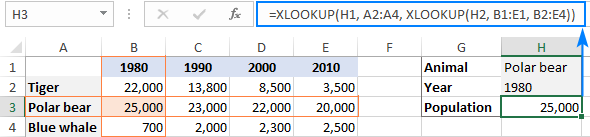Note. The XLOOKUP function is only available in Excel for Microsoft 365, Excel 2021, and Excel for the web.

### How this formula works

The formula uses the ability of XLOOKUP to return an entire row or column. The inner function searches for the target year in the header row and returns all the values for that year (in this example, for year 1980). Those values go to the return_array argument of the outer XLOOKUP:

`XLOOKUP(H1, A2:A4, {22000;25000;700}))`

The outer XLOOKUP function searches for the target animal across the column headers and returns the value in the same position from the return_array.

## SUMPRODUCT formula for two-way lookup

The SUMPRODUCT function is like a Swiss knife in Excel – it can do so many things beyond its designated purpose, especially when it comes to evaluating multiple criteria.

To look up two criteria, in rows and columns, use this generic formula:

SUMPRODUCT(vlookup_column_range = vlookup_value) * (hlookup_row_range = hlookup_value), data_array)

To perform a 2-way lookup in our dataset, the formula goes as follows:

`=SUMPRODUCT((A2:A4=H1) * (B1:E1=H2), B2:E4)`

The below syntax will work too:

`=SUMPRODUCT((A2:A4=H1) * (B1:E1=H2) * B2:E4)`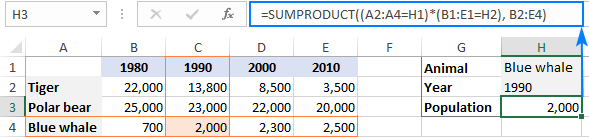### How this formula works

At the heart of the formula, we compare two lookup values against the row and column headers (the target animal in H1 against all animal names in A2:A4 and the target year in H2 against all years in B1:E1):

`(A2:A4=H1) * (B1:E1=H2)`

This results in 2 arrays of TRUE and FALSE values, where TRUE's represent matches:

`{FALSE;FALSE;TRUE} * {FALSE,TRUE,FALSE,FALSE}`

The multiplication operation coerces the TRUE and FALSE values into 1's and 0's and produces a two-dimensional array of 4 columns and 3 rows (rows are separated by semicolons and each column of data by a comma):

`{0,0,0,0;0,0,0,0;0,1,0,0}`

The SUMPRODUCT functions multiplies the elements of the above array by the items of B2:E4 in the same positions:

`{0,0,0,0;0,0,0,0;0,1,0,0} * {22000,13800,8500,3500;25000,23000,22000,20000;700,2000,2300,2500}`

And because multiplying by zero gives zero, only the item corresponding to 1 in the first array survives:

`SUMPRODUCT({0,0,0,0;0,0,0,0;0,2000,0,0})`

Finally, SUMPRODUCT adds up the elements of the resulting array and returns a value of 2000.

Note. If your table has more than one row or/and column headers with the same name, the final array will contain more than one number other than zero, and all those numbers will be added up. As the result, you will get a sum of values that meet both criteria. It is what makes the SUMPRODUCT formula different from INDEX MATCH MATCH and VLOOKUP, which return the first found match.

## Matrix lookup with named ranges (explicit Intersection)

One more amazingly simple way to do a matrix lookup in Excel is by using named ranges. Here's how:

Part 1: Name columns and rows

The fastest way to name each row and each column in your table is this:

1. Select the whole table (A1:E4 in our case).
2. On the Formulas tab, in the Defined Names group, click Create from Selection or press the Ctrl + Shift + F3 shortcut.
3. In the Create Names from Selection dialog box, select Top row and Left column, and click OK.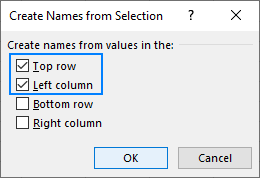This automatically creates names based on the row and column headers. However, there are a couple of caveats:

• If your column and/or rows headers are numbers or contain specific characters that are not allowed in Excel names, the names for such columns and rows won't be created. To see a list of created names, open the Name Manager (Ctrl + F3). If some names are missing, define them manually as explained in How to name a range in Excel.
• If some of your row or column headers contain spaces, the spaces will be replaced with underscores, for example, Polar_bear.

For our sample table, Excel automatically created only the row names. The column names have to be created manually because the column headers are numbers. To overcome this, you can simply preface the numbers with underscores, like _1990.

As the result, we have the following named ranges: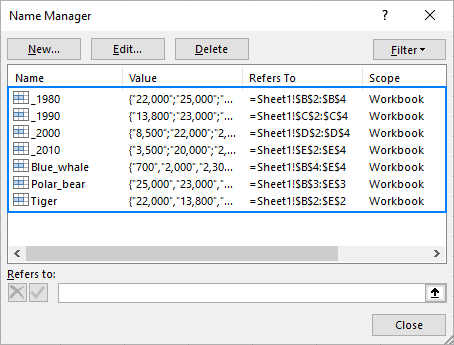Part 2: Make a matrix lookup formula

To pull a value at the intersection of a given row and column, just type one of the following generic formulas in an empty cell:

=row_name column_name

Or vice versa:

=column_name row_name

For example, to get the population of blue whales in 1990, the formula is as simple as:

`=Blue_whale _1990`

If someone needs more detailed instructions, the following steps will walk you through the process:

1. In a cell where you want the result to appear, type the equality sign (=).
2. Start typing the name of the target row, say, Blue_whale. After you've typed a couple of characters, Excel will display all existing names that match your input. Double-click the desired name to enter it in your formula: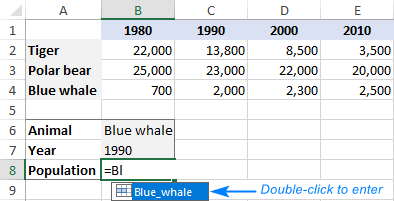3. After the row name, type a space, which works as the intersection operator in this case.
4. Enter the target column name ( _1990 in our case).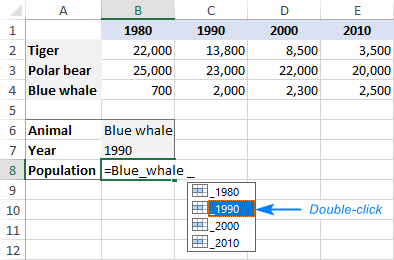5. As soon as both the row and column names are entered, Excel will highlight the corresponding row and column in your table, and you press Enter to complete the formula: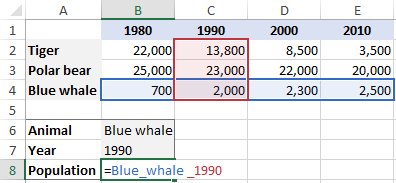Your matrix lookup is done, and the below screenshot shows the result: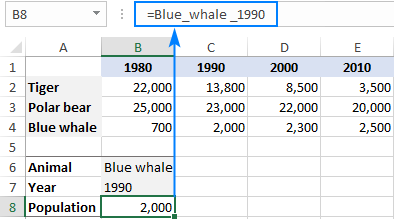That's how to look up in rows and columns in Excel. I thank you for reading and hope to see you on our blog next week!

## You may also be interested in

1. Hello all: I am stressed out surfing internet for solution but can not get to the point. Question is related to XL formula. I need to make following formula working or any other formula you suggest that can do the job would be appreciated. Following formula works but I need to add condition on it:
1. Current formula: INDIRECT("E"&MATCH(B17,INDEX(G:G,A13):INDEX(G:G,B13),0)) ==> works but value it returns is not from my range specified.
2. I need to add condition e.g. when values in a range: INDEX(G:G,A13):INDEX(G:G,B13) > 0 then do rest of work (other words, only consider value greater than 0 in a range). Then I tried following formula:
=INDIRECT("E"&MATCH(B17,IF((INDIRECT("G"& A13):INDIRECT("G"&B13)>0), (INDIRECT("G"& A13):INDIRECT("G"&B13))),0))
This formula also does not test the condition and gives me result that does not fall into specified range.
I am not expert, just trying to learn and putting together the pieces. Using XL 2003

• Hi! I can't check your formulas as I don't have your data and can't guess what results you want.

• Thanks for response. I do see place here to upload the xl file. But will copy & paste data:
Column E: depths with increments by 0.5 e.g. 1.0, 1.5, 2.0 and so on, can to up to 4000 meters.
Columns F, G, H has various types of data that corresponds to depths.
For a certain interval of depths e.g. 1100 to 1190 for this example (but could be any interval within data range).: A15 = 1100, B15= 1190 then
1. First I need find minimum value from Column G with in specified range: which is: 77 ==I am good with this formula
2. then I need to find row number of this value and return relevant cell value from column E which is: E784: 1125.5
but this formula looks in all data and returns me first found number: 77 from column G, E54 = 761.5. (761.5 is not in my specified range but first appearance of number 77 in col G). Please if you can correct this formula or any other version of formula would be appreciated. Again here is formula as specified in first post.
=INDIRECT("E"&MATCH(B17,IF((INDIRECT("G"& A13):INDIRECT("G"&B13)>0), (INDIRECT("G"& A13):INDIRECT("G"&B13))),0))
My data from xl 2007 is:

E: depth, F: data 1, G: data 2, H: data 3
735 -999.25 -999.25 55.90
735.5 -999.25 -999.25 54.50
736 12.6 0 58.51
736.5 11.4 63 55.55
737 11.1 64 53.66
737.5 10.5 57 55.07
738 10.3 57 58.08
738.5 10 63 55.44
739 11.2 66 53.44
739.5 10.5 54 53.74
740 9.5 47 49.30
740.5 8.3 41 55.33
741 7.8 41 55.43
741.5 6.4 41 54.06
742 6 40 49.56
742.5 2.5 40 52.04
743 2.6 43 52.05
743.5 1.7 39 52.90

• Unfortunately, your question is not very clear. Try to explain on a SMALL set of data what you want to do and what result you want to get. You don't need to write a huge number of unnecessary numbers here, as this blog is used by other people as well.

• Column E: depths with increments by 0.5
Columns G has relevant data.
I need to search minimum value (if value greater than zero) from Col: G, from depth range/Col: E; 735 to 740 (A15 = 735, B15= 740). Min value is: 47 from Col: G & then find relevant depth from Col: E, which is: 740. Following formula works good.
=INDIRECT("E"&MATCH(B17,IF((INDIRECT("G"& A13):INDIRECT("G"&B13)>0), (INDIRECT("G"& A13):INDIRECT("G"&B13))),0))
But if I change the search range e.g. Col: E; 740 to 745 (A15 = 740, B15= 745) then my formula fails and gives me wrong result. Resulting minimum value is: 39 and relevant value from Col: E should be: 743.5.
Here my formula fails and gives me wrong answer: 739. I do not know what is wrong with formula changing search range gives me wrong answer. Please let me know if question is still not clear. Here is sample data.
Col: E=Depth Col: G = values
735 -999.25
735.5 -999.25
736 0
736.5 63
737 64
737.5 57
738 57
738.5 63
739 66
739.5 54
740 47
740.5 41
741 41
741.5 41
742 40
742.5 40
743 43
743.5 39
744 53
744.5 58
745 63

• Hi! If I understand your task correctly, try the following formulas:

=INDEX(E1:E30,MATCH(MINIFS(G1:G30,E1:E30,">="&A15,E1:E30,"<="&B15), (E1:E30>=\$A\$15)*(E1:E30<=\$B\$15)*G1:G30, 0))

or

=INDEX(E1:E30,MATCH(MIN(IF((E1:E30>=\$A\$15) * (E1:E30<=\$B\$15)*G1:G30>0,(E1:E30>=\$A\$15) * (E1:E30<=\$B\$15)*G1:G30," ")), (E1:E30>=\$A\$15)*(E1:E30<=\$B\$15)*G1:G30,0))

2. hey, what i want to do is enter 6 values in a single cell // 10,20,30,40,50,60
then I wish to obtain the following result in another cell // =(10*20 + 30*40 50*60)
this needs to be done "without" splitting all the elements in different cells
can you suggest a way to get the result, thanks

• Just an edit in second line
= (10*20)+(30*40)+(50*60)
=4400

• Hi! You can get an array of values that are written as text with the TEXTSPLIT function. You can then select the desired values from this array using the CHOOSECOLS function and do the math calculations. For example:

=CHOOSECOLS(TEXTSPLIT(A1,","),1)*CHOOSECOLS(TEXTSPLIT(A1,","),2) + CHOOSECOLS(TEXTSPLIT(A1,","),3)*CHOOSECOLS(TEXTSPLIT(A1,","),4)

• But what if I don't know the exact count of elements in inside the cell, and only know that they are even in number and need to select odd placed and even placed elements inside the cell?

3. I have a three column table (cells JA7 to JC10) which returns a value based on two criteria of Stage and colour (cells: JA3 to JC4), changing the stage or colour allows for comparisons and in the example below, the three colours are compared on the Stage 1 process.

IZ JA JB JC JE JF

3 Stage S1 S1 S1
4 Colour Yel Blu Grn Min Path

7 Brewery 8 5 4 4 {=INDEX(\$JA\$4:\$JC\$4,MATCH(MIN(IF(\$JA7:\$JC7>0,\$JA7:\$JC7)),\$JA7:\$JC7))}
8 Carpenter 4 4 4 4 {=INDEX(\$JA\$4:\$JC\$4,MATCH(MIN(IF(\$JA8:\$JC8>0,\$JA8:\$JC8)),\$JA8:\$JC8))}
9 Farmer 2 5 4 2
10 Blacksmith 3 14 8 3
And so on...

Column JE returns the minimum value for each item from row 7 onwards.
Column JF has an INDEX MATCH ARRAY formula to return the colour from row 5 based on the lowest value of the relevant line.

The formula is {=INDEX(\$JA\$4:\$JC\$4,MATCH(MIN(IF(\$JA7:\$JC7>0,\$JA7:\$JC7)),\$JA7:\$JC7))}

The problem I have and don't understand how to fix is why when the value in column JC is the lowest (as per line 7), the formula returns a value of #N/A instead of returning a colour from row 4. Whereas all the other results return the relevant "path colour" as the result.

• Hi! Notice that you didn't use the Match_type argument in the MATCH function. Therefore, you are using an approximate match, in which the data must be sorted in ascending order. Use 0 for the Match_type argument. Read more in this guide: How to use MATCH function in Excel.

4. I am trying to do something similar but a little backwards from what is describes here. Please help.

Background: We use a spreadsheet as a calendar schedule at work. Names are in the first column and Dates (01 Jan - 31 Dec) are the first row. In the second row are the names of specific events. Each row/column intersection is marked with the type of work that was performed by each name on the given day. For the purposes here, I'll call the events "X" and "Y" work types "A" and "B".

I'll try to make a little example here:

1 2 3 4 5
Event X X X
Bob A B B
Joe A B

I need to pull the names (column A) of anybody that performed any work (A or B) during event X.

I tried using something along the lines of INDEX(Names, MATCH(1, (Events=X)*(OR(WorkDaysArray=A,WorkDaysArray=B)), 0),) but the WorkDaysArray is a 2-dimensional array that will apparently not work within a MATCH formula. I'm stumped.

I have already implemented a second sheet that automatically lists all of the events in one column, starting date, number of days, and ending date using INDEX and MATCH formulas. I just can't figure out this last piece, which should list all Names that worked a given event in some additional columns.

So following my example, I would want the output to look something like this:

Event Name1 Name2
X Bob Joe

• Well my spaces in the example were removed but hopefully y'all still get the idea. I'll try again without using spaces:

Date____1_____2_____3_____4_____5
Event_________X_____X_____X______
Bob_____A____B__________________B
Joe__________________A_____B______
Sam____A________________________B

I am trying to develop a formula that will output "Bob" and "Joe" but not "Sam" into a separate sheet like this:

Event__Start__#days__End__Name1__Name2__Name3
X_______2______3_______4____Bob______Joe______________
Y________________________________________________________

• Hello!
Your request goes beyond the advice we provide on this blog. This is a complex solution that cannot be found with a single formula. If you have a specific question about the operation of a function or formula, I will try to answer it.

The Excel is all about scheduling the crews in different jobs, I have two sheets in the spreadsheet, Sheet 1 is the Manpower Schedule wherein you find the names of the crew with dates in columns, like column A is intended for the names and Columns B to E are the dates. Sheet 2 is the Planned Job Schedule wherein you find the Jobs in column A and columns B to E are the dates.

This is my problem. I can't figure out how to automate the process if there are two names indicated in a job. It cannot be detected that the person will be working on that job.

I figured out how to automate if the match is the exact name. and this is the formula that I made. =IF(COUNTIF('Planned Job Schedule'!B\$3:B350,'Manpower Schedule'!\$A3)>1,"Multiple Jobs",IFERROR(INDEX('Planned Job Schedule'!\$A\$3:\$A50,MATCH(\$A3,'Planned Job Schedule'!B\$3:B100,0),1),""))

Sheet 2:
Job December 3 December 4 December 5
Wall Isolation Ronald Ronald, Alfredo

Sheet 1
Name December 3 December 4 December 5
Ronald Wall Isolation
Alfredo

• Sheet 2:
The job is Wall Isolation and on December 3 only Ronald is working while on December 4 both Ronald and Alfredo are working

Sheet 1:
The job appeared in Column December 3 since it only entered one name, while on December 4, nothing appeared since there are two names entered.

6. Hi, I'm trying to do the reverse of what this document is doing, (the example is that for a specific valve size a certain valve flow co-efficient (Cv) is calculated and for the given Cv a degree of opening is given.
I want to use the valve size on the y axis and the Cv the range of values in the array to give me the value that corresponds to the two meeting values in the row at the top

• Hi!
As it's currently written, it's hard to tell exactly what you're asking. Please describe your problem in more detail.

7. Hello,

Does anyone know how to match 2 columns so that the numbers in column 'A' (e.g. key) match within their rows to the numbers in column 'B' (e.g. ID), while keeping everything from columns C (videos, number of views) onwards aligned to the current column 'B'?

key, ID, no. videos, number of views
3 10 8 20
10 27 2 8
25 3 4
27 5 1

Many thanks

Amber

• Hi!
Based on your description, it is hard to completely understand your task. However, I’ll try to guess and offer you the following formula:

=IFERROR(INDEX(B1:D4,MATCH(A1,B1:B4,0),),"")

How to get all the values in a string using the INDEX function, read this guide.

8. Hi, I'm hoping someone can assist me with my training matrix formula please?

I have two worksheets. One has the report from our training system which has the candidates names along row 1 and the courses down column B. In row 1 there are duplicate candidate names as the report pulls from multiple roles, I am trying to drag that information (their training status) into another worksheet from the intersecting cells.

I have tried the Index-Match-Match and the X-Lookup though I get an error for one and 0 for the other, which may be due to the duplicate names.

What can you suggest?

• Hi!
Your task is not completely clear to me. Explain what result you want to get.

• Hi Alexander,

I want to return the training status (competent, expired, etc) for a candidate against each of the required courses for their role from a report I have downloaded.

Sheet1 B2:B36 lists the courses I want to match in Sheet 2

Sheet1 C1:Z1lists the candidates names I want to match in Sheet2
Sheet2 B1:EN1 lists the candidates names from the report I have downloaded.

Example: I want to match B2 & C1 from sheet1 in sheet2 and return the text in the intersecting cell if available (blank, if nothing)
Issue: C1 has duplicate matches in sheet2 from B1:N1 though only one value in row 2 for that course.

Let me know if you require any further information.

Many thanks,
Jacinta

• Hello!
To do a two-way lookup and extract all values from duplicate column headings, try this formula:

=TEXTJOIN(", ",TRUE,IFERROR(INDEX(Sheet2!\$B\$2:\$G\$6, MATCH(Sheet1!B2,Sheet2!\$A\$2:\$A\$6,0), IF((Sheet2!\$B\$1:\$G\$1=Sheet1!C1),(COLUMN(Sheet2!\$B\$1:\$G\$1)-1),"")),""))

The TEXTJOIN function merges all found results in one cell.
Hope this is what you need.

• Thanks Alexander,

That's an impressive formula :)

How do I return the results from only the cell that has text in it and disregard all blank cells?

• Hello!
Add the TEXT function to the formula to hide zero values. Numeric formats for displaying zero as a blank can be found here.

=TEXTJOIN(", ",TRUE,TEXT(IFERROR(INDEX(Sheet2!\$B\$2:\$G\$6, MATCH(Sheet1!B2,Sheet2!\$A\$2:\$A\$6,0), IF((Sheet2!\$B\$1:\$G\$1=Sheet1!C1),(COLUMN(Sheet2!\$B\$1:\$G\$1)-1),"")),""),"#,##0;-#,##0;"))

• Thank you.
I have entered this: =TEXTJOIN(", ",TRUE,TEXT(IFERROR(INDEX(Report!\$B\$2:\$JG\$219, MATCH(\$B2, Report!\$A\$2:\$A\$219,0), IF((Report!\$B\$1:\$JG\$1=[Sheet1]Competency!C\$1),(COLUMN(Report!\$B\$1:\$JG\$1)-1),"")),""),"#,##0;#,##0;"))

It only works in the first column? I have copy and pasted to the rest of the worksheet and the rest come up blank.

Some cells in the first column are bring back duplicate values also, is there a way to remove duplicate values?

Many thanks,

• Hi!
You can also find useful information in this article: How to copy formula in Excel with or without changing references.
Try to use Sheet1!\$B2 and Sheet1!C\$1 -

=TEXTJOIN(", ",TRUE,TEXT(IFERROR(INDEX(Sheet2!\$B\$2:\$G\$6, MATCH(Sheet1!\$B2,Sheet2!\$A\$2:\$A\$6,0), IF((Sheet2!\$B\$1:\$G\$1=Sheet1!C\$1),(COLUMN(Sheet2!\$B\$1:\$G\$1)-1),"")),""),"#,##0;-#,##0;"))

9. In the formula below, A33:A500 is a list of sorted dates: older at top.
E33:E500 is a list of balances for dates in A33:A500.
B2 is current Date, D33:D500 is a list of varies descriptions.
In the formula we are looking for the same, one “Unique description” in both places in the formula.

The formula is working as expected without any issues.
The result is a balance amt. from E33:E500.

I was wondering if “XLOOKUP” could replace (and would be a good fit for) Index/match, both times in this formula?
And how would the formula be written?

Thanks
Joe

{=MIN(INDEX(A33:E500,MATCH(1,(B2<=A33:A500)*(D33:D500="Unique description"),0),5):INDEX(A33:E500,MATCH(1,(14+B2<=A33:A500)*(D33:D500="Unique description"),0),5))}

10. Hi, if i have the approximate population, i want it return in the year...May i know what is the formula?

• Hi!
I am not sure I fully understand what you mean.

11. Hey,
You're an amazing help and make Excel super easy to understand so thank you!!
I just have one question...I work as an office manager for a bar and I'm trying to collect all my waiters/bartenders tips onto one page. Every night they write down on separate sheets (same workbook) how many tips they got and I want to be able to find them all in one easy table. How do I use IndexMatch across multiple sheets without having to rewrite the formula for each person and for each day?
The different sheets are labeled by the day of the month literally 1,2,3,4 etc through 31
Thank you so much!! You're so helpful!!
Alexa

12. Hi, can you advise how to do a 2-way lookup in a table of numbers where the row and column headers are also numbers? So by inputting a number for the row header, and another number for the column headers, it would return a number from the table. Where the input row and column header numbers are not exactly matching the header number, it will look to the next higher header number and return the matrix number. Is that possible?

• Hello!
If the number of row and column headings are in descending order, then you can use the formula:

=INDEX(B2:E4, MATCH(H1,A2:A4,-1), MATCH(H2,B1:E1,-1))

13. This is a great summary of all available methods for 2d lookups. Which method is the fastest for exact lookups?

14. Hi,
You've been the best Excel resource ive found!
Hope you can help this time too.
First time writing.

This is one of the formulas I have on top of my checking acct. registrar:

F13=
=SUMIF(INDEX(D33:D1000,MATCH(F10,A33:A1000,1),):INDEX(D33:D1000,MATCH(F12,A33:A1000,1),),F4,IF(F2="Dept",INDEX(A33:D1000,MATCH(F10,A33:A1000,1),2):INDEX(A33:D1000,MATCH(F12,A33:A1000,1),2),IF(F2="Credit",C33:C1000,0)))

Columns:
Date=A
Dept=B
Credit=C
Description=D
Running Balance=E

Inputs: (F2,F4,F10,F12)
F4= A repetitive Description entry ("Electric Bill","Income")
Date Range= Start date=F10, End date=F12

Output:F13

Data Rows= @ 33:1000

The dates are ascending in Column A, but because the dates aren't daily sequential, the formula results in using a date before(< F10) Because of the MATCH TYPE Rules!

When F10 Date exist the formula works fine, its only when F10 date doesn't exist the formula uses a date prior to that of F10! (MATCH TYPE Rules)

Do you know how I can correct the formula so that if F10 date doesn't exist, the formula will start evaluating the next available match/Date in Column A after F10?

Thanks!
Joe

• Hello!
Unfortunately, without seeing your data it is difficult to give you any advice.
When using parameter 1 in the MATCH function, the data must be sorted. About your phrase "The dates are ascending in Column A, but because the dates aren't daily sequential" I cannot say that.
I can also suggest using a search by date range from F10 to F12. Pay attention to this article - INDEX MATCH with several criteria.

15. A B C D E
A 34 34 34 34 34
B 54 54 54 54 54
C 12 11 10 9 8
D 7 6 5 4 3
E 2 1 15 8 7

I have this table, I want the value from this table when I enter AC it will give me 34. if there is any formula please let me know.

• Hello!
I kindly ask you to have a closer look at the following paragraph of the article above.

• C D E F G H House 1 House 2
1 3 5 7 9 11 13 15 17 19
-------------------------------------
8 44 54 58 59 86
27 29 58 64 67 80
5 10 47 59 66 73
22 23 24 59 63 81
11 12 44 57 77 89
2 11 15 39 84 87
18 22 31 54 71 80
2 5 7 12 23 42
9 10 36 67 76 83
2 13 22 37 48 90
6 9 29 41 50 90
9 16 19 26 28 46
16 37 48 68 84 90
14 26 31 40 58 68

Dear Team,
I am Sharing my Problem to your Good self Kindly Help me to Solve .
On the Left side of Table I have some Winning Numbers under the Alphabet Columns
These are 14 Draws Held in a Month.

On Right side are the Odd Numbers HOUSE 1(1,3,5,7,9) and HOUSE2(11,13,15,17,19)
My Goal is to Shift/Fill numbers from Left side i.e Draw to the Houses on Right side.

How can i do with Command or Which Command can solve my Problem I tried several but no satisfactory Outcome achieved

Regards
Mehtaab.

• Hi!
Sorry, it's not quite clear what you are trying to achieve.

16. Thanks for this it was really helpful

17. Hi Svetlana,
How can I find row title based on the matrix value. In you Example I want to find row title for D3 (22,000) or E4 (2500). I need a reverse formula. It means I have the year and population but need to know where they belong to, Tiger, Polar bear,..
How can I solve it.
Ali

18. Thank you! I’ve looking for the index match match. Beautifully and easily put together.

19. Can you please advise how we can drag the results down to apply the formulae to multiple rows in a sheet.

I tried double clicking the square and also the copy pste special formulae in mass but does not seem to update the value until we double click in the formula itself.

Thank you,

Nikhil

20. hi need help with this
with the assigned value like a=1 , s=2, d=3, f=4